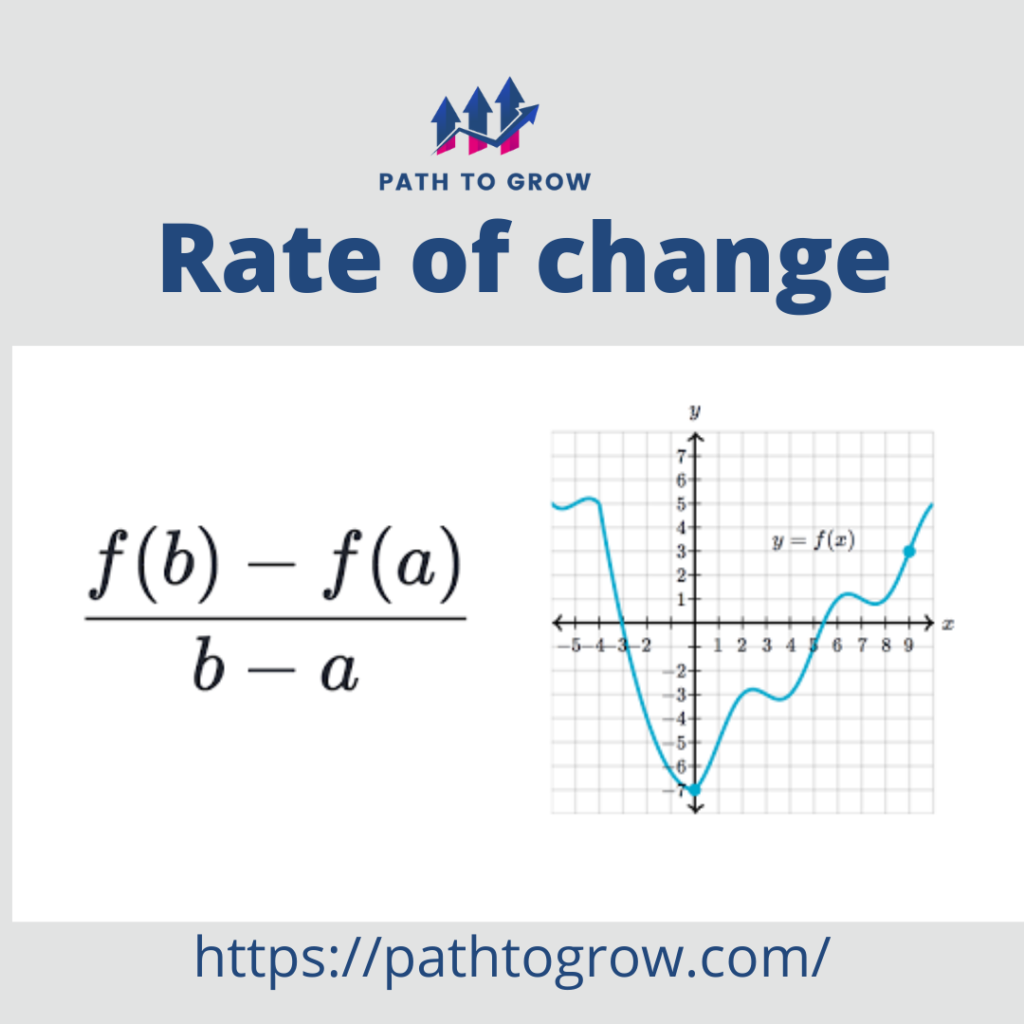# Marginal Rate of Substitution## What is the Rate Of Change Formula? – Calculation and Application

What is the Rate Of Change Formula? – Calculation and Application The rate of change function is calculated by dividing the amount of change in one item with respect to another. So, it can be defined as the amount of change in a quantity with respect to another. The rate of change formula can be …